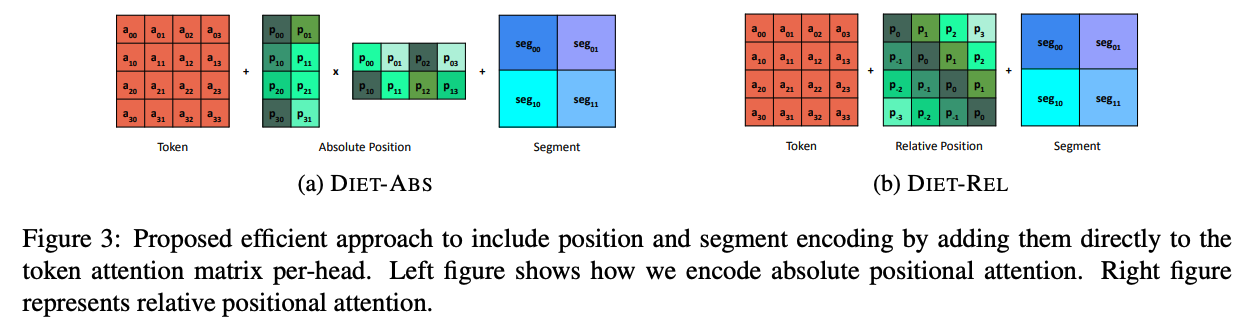# 简单有效的位置编码$$\newcommand{\R}{\mathbb{R}} \def\rmA{{\mathbf{A}}} \def\rmB{{\mathbf{B}}} \def\rmC{{\mathbf{C}}} \def\rmD{{\mathbf{D}}} \def\rmE{{\mathbf{E}}} \def\rmF{{\mathbf{F}}} \def\rmG{{\mathbf{G}}} \def\rmH{{\mathbf{H}}} \def\rmI{{\mathbf{I}}} \def\rmJ{{\mathbf{J}}} \def\rmK{{\mathbf{K}}} \def\rmL{{\mathbf{L}}} \def\rmM{{\mathbf{M}}} \def\rmN{{\mathbf{N}}} \def\rmO{{\mathbf{O}}} \def\rmP{{\mathbf{P}}} \def\rmQ{{\mathbf{Q}}} \def\rmR{{\mathbf{R}}} \def\rmS{{\mathbf{S}}} \def\rmT{{\mathbf{T}}} \def\rmU{{\mathbf{U}}} \def\rmV{{\mathbf{V}}} \def\rmW{{\mathbf{W}}} \def\rmX{{\mathbf{X}}} \def\rmY{{\mathbf{Y}}} \def\rmZ{{\mathbf{Z}}}$$

那么$\rmA_a = (\rmX+\rmP) \rmW_Q \rmW_K^\top (\rmX+\rmP)^\top$就是加到输入上产生的注意力矩阵（忽略缩放等小细节）了。根据秩的性质，它的秩的上界是受限于每个注意力头的大小的：
\begin{align*} rank(\rmA_a) &= rank((\rmX+\rmP) \rmW_Q \rmW_K^\top (\rmX+\rmP)^\top) \\ &\le \min(rank(\rmX+\rmP), rank(\rmW_Q), rank(\rmW_K)) \\ &\le d_h. \end{align*}

而加到注意力矩阵时产生的新注意力矩阵为$\rmA_r = \rmX \rmW_Q \rmW_K^\top \rmX^\top + \hat{\rmP} \hat{\rmP}^\top$，它的秩可以通过构造法来得到。构造$\rmW_Q = \rmW_K$为前$d_h$行是单位矩阵、其他行为$0$。则两者乘积为：
$$\rmW_Q \rmW_K^\top = { \left( \begin{array}{cc} I_{d_h, d_h} & 0_{d_h, d-d_h}\\ 0_{d-d_h, d_h} & 0_{d-d_h, d-d_h} \end{array} \right) }$$

$$\rmX \rmW_Q \rmW_K^\top \rmX^\top = { \left( \begin{array}{cc} I_{d_h, d_h} & 0_{d_h, n-d_h}\\ 0_{n-d_h, d_h} & 0_{n-d_h, n-d_h} \end{array} \right) }$$

$$\hat{\rmP} \hat{\rmP}^\top = { \left( \begin{array}{cc} 0_{n-d_p, n-d_p} & 0_{n-d_p, d_p}\\ 0_{d_p, n-d_p} & I_{d_p, d_p} \end{array} \right)}$$

\begin{align*} rank(\rmA_r) &= rank(\rmX \rmW_Q \rmW_K^\top \rmX^\top + \hat{\rmP} \hat{\rmP}^\top) \\ & = min(d_h + d_p, n) > d_h. \end{align*}